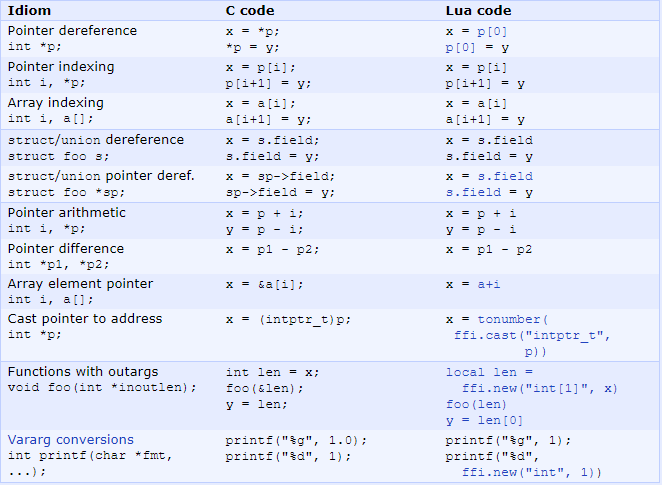# 1. FFI 教程

### 加载 FFI 库

FFI 库时默认编译进 LuaJIT 中的，但是不会默认加载或初始化。因此，当需要使用 FFI 库时，需要在 Lua 文件的开头添加如下语句：

local ffi = require("ffi")


### 访问标准系统函数

local ffi = require("ffi")
ffi.cdef[[
void Sleep(int ms);
int poll(struct pollfd *fds, unsigned long nfds, int timeout);
]]

local sleep
if ffi.os == "Windows" then
function sleep(s)
ffi.C.Sleep(s*1000)
end
else
function sleep(s)
ffi.C.poll(nil, 0, s*1000)
end
end

for i = 1, 160 do
io.write("."); io.flush()
sleep(0.01)
end
io.write("\n")


### 访问 zlib 压缩库

local ffi = require("ffi")
-- 定义由 zlib 提供的 C 函数
ffi.cdef[[
unsigned long compressBound(unsigned long sourceLen);
int compress2(uint8_t *dest, unsigned long *destLen,
const uint8_t *source, unsigned long sourceLen, int level);
int uncompress(uint8_t *dest, unsigned long *destLen,
const uint8_t *source, unsigned long sourceLen);
]]
-- 加载 zlib 共享库。在 POSIX 系统上，名为 libz.so，通常是预安装的。
-- 因为 ffi.load() 会自动添加缺失的标准前缀/后缀，因此可以简单地加载 "z" 库。
local zlib = ffi.load(ffi.os == "Windows" and "zlib1" or "z")

local function compress(txt)
-- 首先，通过使用未压缩字符串的长度来调用 zlib.compressBoud 来获取
-- 压缩缓存区的最大大小.
local n = zlib.compressBound(#txt)
-- 分配这个 n 大小的字节缓存区，类型规范中的 [?] 表示可变长度数组(VLA).
-- 该数组的实际元素个数由 ffi.new 的第二个参数给出.
local buf = ffi.new("uint8_t[?]", n)
-- 看上面 compress2 的函数声明可知，destLen 被定义为一个指针。这是因为
-- 传入的是最大缓存区的大小并返回实际使用的长度.
-- 在 C 中可以通过传入一个本地变量的地址 (即 &buflen)，但是在 Lua 中没有
-- 地址操作，因此传入的是只有一个元素的数组。
local buflen = ffi.new("unsigned long", n)
local res = zlib.compress2(buf, buflen, txt, #txt, 9)
assert(res == 0)
-- 将压缩数据作为 Lua 字符串返回，因此使用 ffi.string()，它需要指向
-- 数据开头和实际长度的指针，这个长度已经通过 buflen 数组返回了
return ffi.string(buf, buflen)
end

local function uncompress(comp, n)
local buf = ffi.new("uint8_t[?]", n)
local buflen = ffi.new("unsigned long", n)
local res = zlib.uncompress(buf, buflen, comp, #comp)
assert(res == 0)
return ffi.string(buf, buflen)
end

-- Simple test code.
local txt = string.rep("abcd", 1000)
print("Uncompressed size: ", #txt)
local c = compress(txt)
print("Compressed size: ", #c)
local txt2 = uncompress(c, #txt)
assert(txt2 == txt)


### 为 C Type 定义 Metamethods

local ffi = require("ffi")
ffi.cdef[[
typedef struct { double x, y; } point_t;
]]

local point
local mt = {
__add = function(a, b) return point(a.x+b.x, a.y+b.y) end,
__len = function(a) return math.sqrt(a.x*a.x + a.y*a.y) end,
__index = {
area = function(a) return a.x*a.x + a.y*a.y end,
},
}
point = ffi.metatype("point_t", mt)

local a = point(3, 4)
print(a.x, a.y) --> 3  4
print(#a)       --> 5
print(a:area()) -- 25
local b = a + point(0.5, 8)
print(#b)       --> 12.5


### C 和 LuaJIT 相互转化### 缓存或不缓存

local byte, char = string.byte, string.char
local function foo(x)
return char(byte(x) + 1)
end


local funca, funcb = ffi.funca, ffi.C.funcb -- Not helpful
local function foo(x, n)
for i = 1, n do funcb(funca(x, i), 1) end
end


local C = ffi.C         -- Instead use this
local function foo(x, n)
for i = 1, n do C.funcb(C.funca(x, i), 1) end
end


# 2. ffi.* API

#### 词汇表

• cdecl：抽象 C 类型声明（Lua 字符串）。
• ctype：C 类型对象。由 ffi.typeof() 返回的一种特殊的 cdata，当被调用时是作为 cdata 的构造函数。
• ct：一种类型规范，可用于大多数 API 函数。cdecl，ctype 或 cdata 作为模板类型。
• cb：一个回调对象。这是一个包含特殊函数指针的 C 数据对象。从 C 代码调用此函数会运行关联的 Lua 函数。
• VLA：通过 [?] 代替元素个数值声明的一个可变长度数组，如 "int[?]"。当创建的时候必须给出元素个数。
• VLS：可变长度结构体是一个 C 类型的结构体，最后一个元素是 VLA。适用于声明和创建的相同规则。

## 2.1 声明和访问外部符号

### 2.1.1 ffi.cdef(def)

ffi.cdef[[
typedef struct foo { int a, b; } foo_t;  /* Declare a struct and typedef. */
int dofoo(foo_t *f, int n);              /* Declare an external C function */
]]


##### 如何使用自定义的函数？

int add(int x, int y)
{
return x + y;
}


gcc -g -o libmyffi.so -fpic -shared myffi.c


export LD_LIBRARY_PATH=\$LD_LIBRARY_PATH:your_lib_path


ffi.load(name, [,global])


ffi.load 会通过给定的 name 加载动态库，返回一个绑定到这个库符号的新的 C 库命名空间，在 POSIX 系统中，如果 global 被设置为 true，这个库符号被加载到一个全局命名空间。另外这个 name 可以是一个动态库的路径，那么会根据路径来查找，否则的话会在默认的搜索路径中去找动态库。在 POSIX 系统中，如果在 name 这个字段中没有写上点符号 .，那么 .so 将会被自动添加进去，例如 ffi.load("z") 会在默认的共享库搜寻路径中去查找 libz.so，在 windows 系统，如果没有包含点号，那么 .dll 会被自动加上。

local ffi = require("ffi")

ffi.cdef[[
int add(int x, int y); /* don't forget to declare */
]]

print(res)  -- output: 3   Note: please use luajit to run this script.


local ffi = require"ffi"

ffi.cdef[[
int add(int x, int y);   /* don't forget to declare */
]]

print(res) -- output: 3   Note: please use luajit to run this script.


## 2.2 创建 cdata 对象

### 2.2.1 ffi.typeof

ctype = ffi.typeof(ct)


local uintptr_t = ffi.typeof("uintptr_t")
local c_str_t = ffi.typeof("const char*")
local int_t = ffi.typeof("int")
local int_array_t = ffi.typeof("int[?]")


### 2.2.2 ffi.new

cdata = ffi.new(ct [,nelem] [,init...])
cdata = ctype([nelem,] [init...])


ffi.new 开辟空间，第一个参数为 ctype 对象，ctype 对象最好通过 ctype = ffi.typeof(ct) 构建。

#### ffi.new 和 ffi.C.malloc 的区别？

local int_array_t = ffi.typeof("int[?]")
local bucket_v = ffi.new(int_array_t, bucket_sz)

local queue_arr_type = ffi.typeof("lrucache_pureffi_queue_t[?]")
local q = ffi.new(queue_arr_type, size + 1)


### 2.2.3 ffi.cast

cdata = ffi.cast(ct, init)


local c_str_t = ffi.typeof("const char*")
local c_str = ffi.case(c_str_t, str)  -- 转换为指针地址

local uintptr_t ffi.typeof("uintptr_t")
tonumber(ffi.cast(uintptr_t, c_str)   -- 转换为数字


### 2.2.4 ffi.metatype

ctype = ffi.metatype(ct, metatable)


posted @ 2018-08-04 10:08  季末的天堂  阅读(1216)  评论(0编辑  收藏